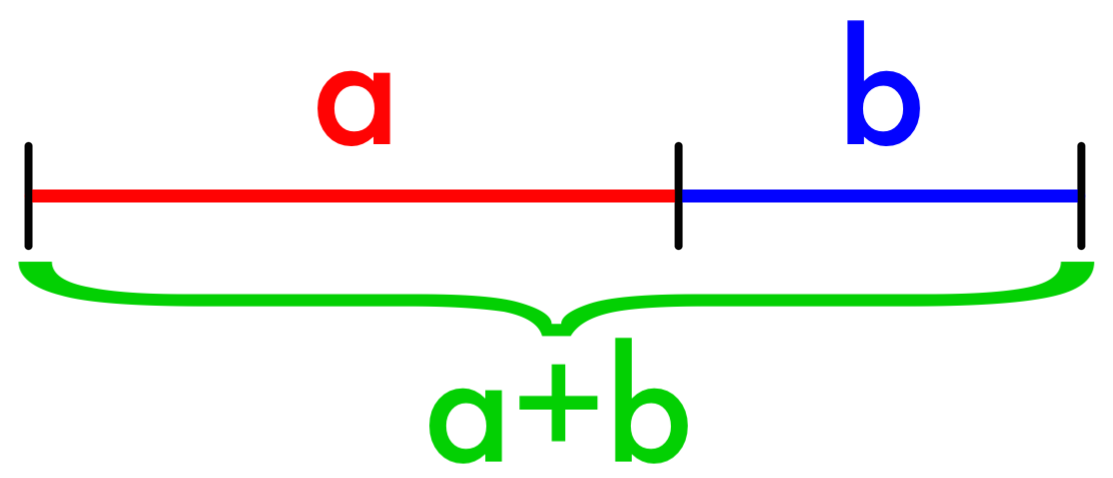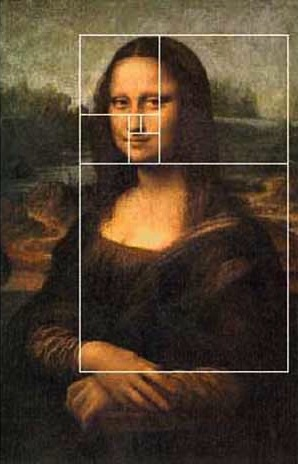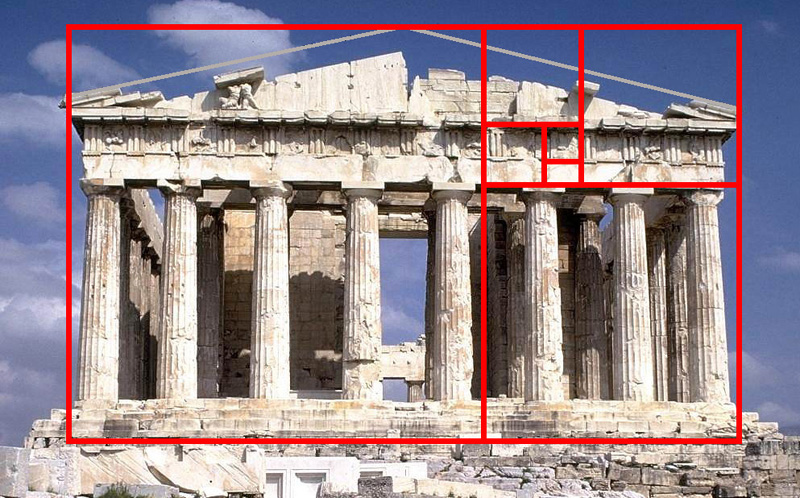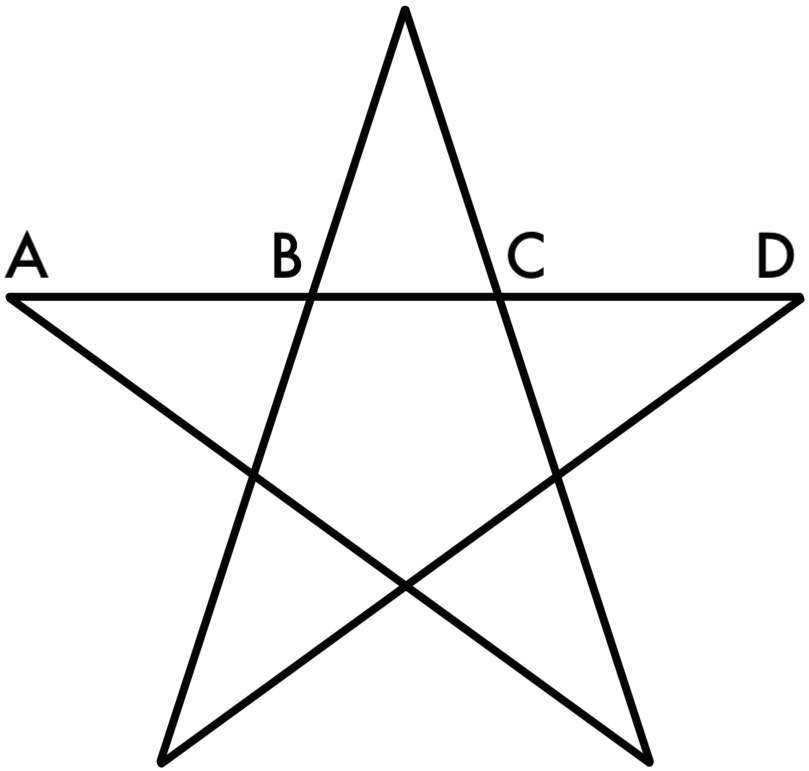# The Golden Ratio

The Golden Ratio is a very beautiful mathematical topic in many ways. For one thing, it has long been used to analyze our sense of beauty. For another, it’s a very beautiful topic mathematically.The Golden Ratio is often defined as the ratio a/b, where the ratio of a to b is the same as the ratio of the entire segment to a. Green is to red as red is to blue.We can use this definition to get the value of the golden ratio – all it takes is a little algebra. I won’t go through all the steps here, but suffice it to say that you can use this definition to set up a proportion and solve to find that the Golden Ratio is equal to

$$\dfrac{1 + \sqrt{5}}{2} \approx 1.618$$

Well, this isn’t terribly illuminating. But we can understand this better if we take this definition from one dimension to two. There’s a rectangular version of the Golden Ratio called (appropriately) the Golden Rectangle.Here’s how it works: The middle line chops the big rectangle in two so the left thing is a square and the right thing is a smaller rectangle. The Golden Rectangle happens when the proportions of the little rectangle are the same as the proportions of the big rectangle.

Why is this the same as the ratio we defined before? Well, if we call the side length of the square “a,” and width of the small rectangle “b,” then the length of the big rectangle is a+b. Then the proportions of the big rectangle are a+b to a, and the proportions of the little rectangle are a to b. Green is to red as red is to blue.This definition means that the small rectangle has the same proportions as the big rectangle – in other words, it’s another Golden Rectangle. That means we can chop it up as well, into a square and a smaller Golden Rectangle.And since the new little rectangle is also a Golden Rectangle, we can chop it up too.And we can keep going as long as we want.What’s more, we can draw quarter circles in all the squares:And we get a spiral.

Well now. Those of you who read the page on Fibonacci number patterns might notice that this looks a whole lot like the Fibonacci Spiral. In fact, both spirals are very very very good approximations of a logarithmic spiral. A logarithmic spiral would pass through all the same corners that our quarter circles do, but it would change its curvature smoothly instead of hopping from quarter circle size to quarter circle size.A nautilus shell (like the one above) grows in a logarithmic spiral, always getting bigger but keeping the same proportions, much like this golden spiral grows. You can find many other examples of logarithmic spirals in nature. And that’s not the only way the Golden Ratio appears in nature – check out its close cousin the Golden Angle.

You can also find intriguing parallels to the Golden Ratio in many pieces of art, often using the Golden Rectangle. Some people claim that the Golden Ratio is hardwired into our sense of aesthetic as the most beautiful proportion and therefore shows up in such places as the Mona Lisa and the Parthenon.Is this true, or is it just hype? Frankly, nobody knows for certain. It’s still a mystery how much the Golden Ratio is really tied to our sense of beauty. At the very least its artistic parallels have fascinated and inspired people through the ages. But one thing is for certain: mathematically speaking, it deserves its name.

For instance, take the pentagram, a five-pointed star made of five straight lines.This shape is riddled with Golden Ratios. For example: AD is to AC, as AC is to CD, as CD is to BC. The more you look for it, the more you find it!

But that’s not all! If you look closely, you’ll find it in yet another surprising place